# A string is fixed at both ends. The mass of the string is 0.0010 kg and...

A string is fixed at both ends. The mass of the string is 0.0010 kg and the length is 2.4 m. The string is under a tension of 210 N. The string is driven by a variable frequency source to produce standing waves on the string. Find the wavelengths and frequencies of the first four modes of standing waves. Express all wavelengths rounded to two decimal places and all frequencies rounded to one decimal place.

 λ1=____ m λ2=___ m λ3=_____ m λ4=_____ m f1=___ Hz f2=___ Hz f3=_____ Hz f4=_____ Hz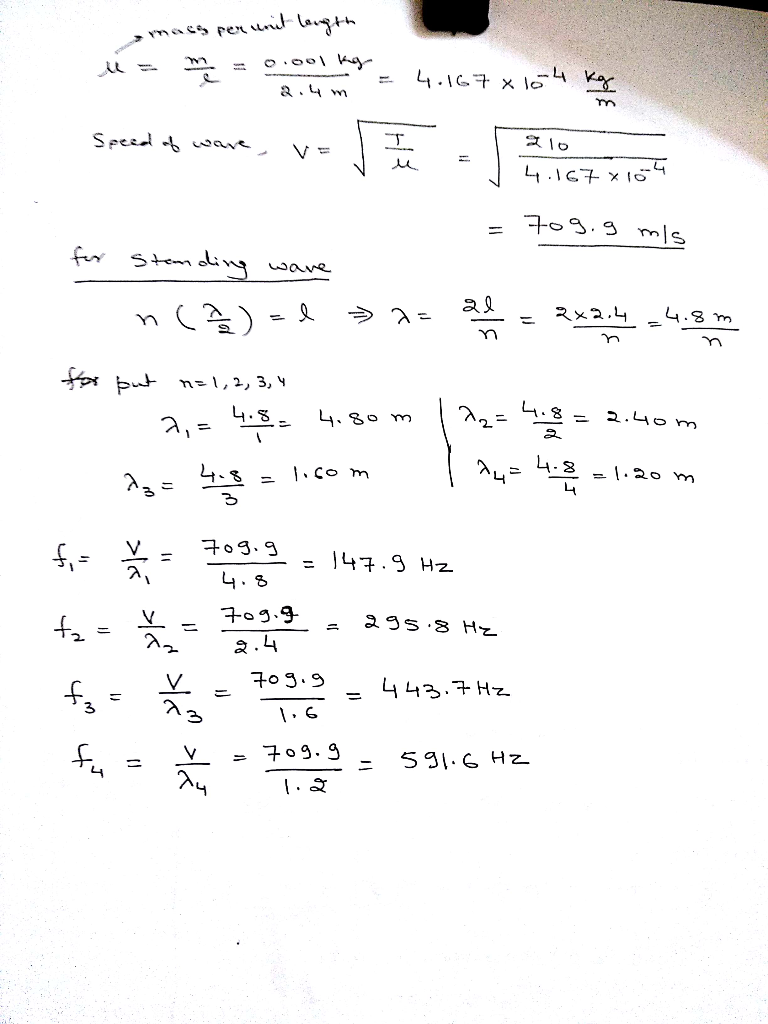#### Earn Coin

Coins can be redeemed for fabulous gifts.

Similar Homework Help Questions
• ### A 2.8 m long wire having a mass of 0.14 kg is fixed at both ends...

A 2.8 m long wire having a mass of 0.14 kg is fixed at both ends and is under tension of 37 N. When the nth harmonic is excited, there is a node 0.56 m from one end. (a) What is n? n = (b) What are the frequencies of the first three allowed modes of vibration? f1 = Hz f2 = Hz f3 = Hz

• ### (27) Standing waves on a 1.5-meter long string that is fixed at both ends are seen...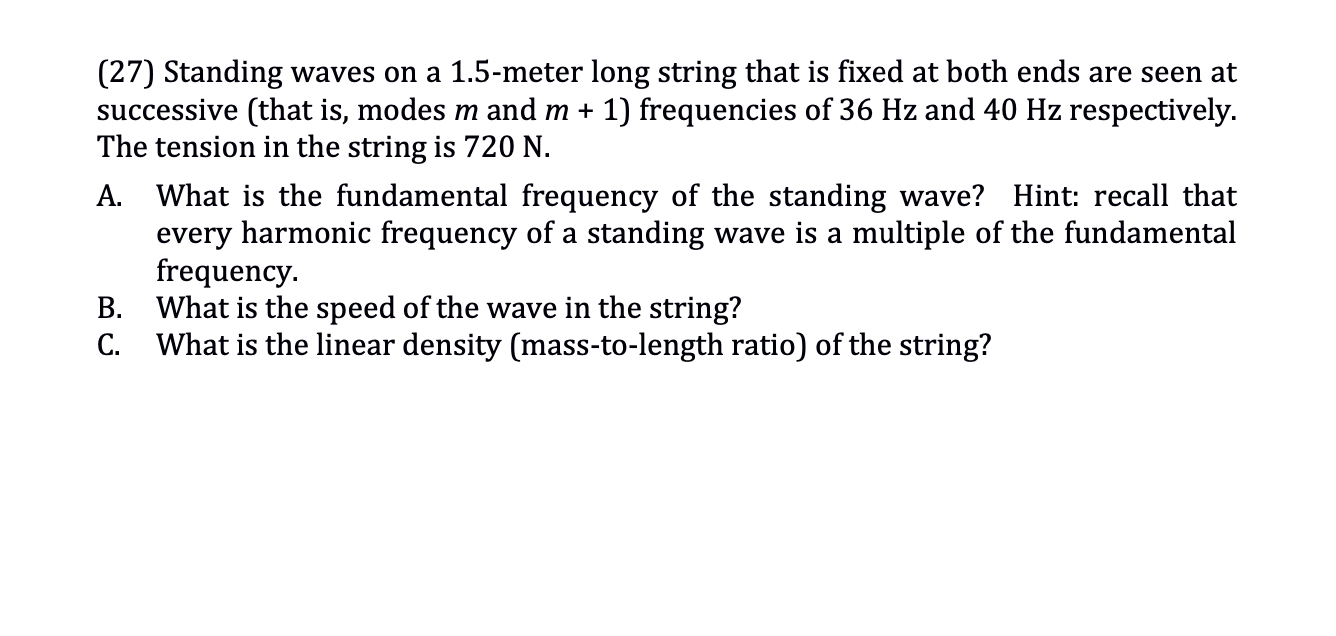(27) Standing waves on a 1.5-meter long string that is fixed at both ends are seen at successive (that is, modes m and m + 1) frequencies of 36 Hz and 40 Hz respectively. The tension in the string is 720 N. A. What is the fundamental frequency of the standing wave? Hint: recall that every harmonic frequency of a standing wave is a multiple of the fundamental frequency B. What is the speed of the wave in the string?...

• ### (27) Standing waves on a 1.5-meter long string that is fixed at both ends are seen...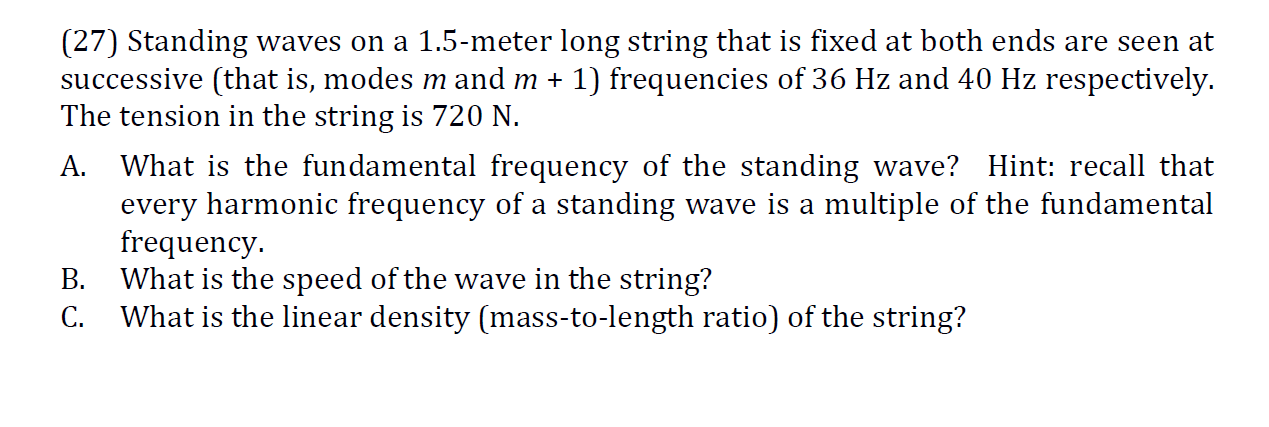(27) Standing waves on a 1.5-meter long string that is fixed at both ends are seen at successive (that is, modes m and m + 1) frequencies of 36 Hz and 40 Hz respectively. The tension in the string is 720 N. A. What is the fundamental frequency of the standing wave? Hint: recall that every harmonic frequency of a standing wave is a multiple of the fundamental frequency. B. What is the speed of the wave in the string?...

• ### (5 points) When a string that is fixed at both ends is plucked, it can vibrate...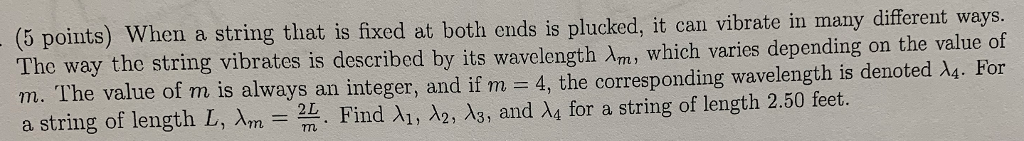(5 points) When a string that is fixed at both ends is plucked, it can vibrate in many different ways The way the string vibrates is described by its wavelength λ which varies depending on the value of m. The value of m is always an integer, and if m 4, the corresponding wavelength is denoted λ4. For a string of length L. Arn-24. Find λι, λ2, λ3, and λ4 for a string of length 2.50 feet.

• ### A string fixed at both ends is 8.60 m long and has a mass of 0.118 kg. It is subjected to a...

A string fixed at both ends is 8.60 m long and has a mass of 0.118 kg. It is subjected to a tension of 90.0 N and set oscillating.(A) What is the speed of the waves on the string? ____ m/s(B) What is the longest possible wavelength for a standing wave? _____ m(C) Give the frequency of that wave. _____ HzThank you :)

• ### A string fixed at both ends has successive resonances with wavelengths of 0.55 m for the...

A string fixed at both ends has successive resonances with wavelengths of 0.55 m for the nth harmonic and 0.53 m for the (n + 1)th harmonic. (a) What are the following values? nth harmonic (n + 1)th harmonic (b) What is the length of the string? m Use the fact that the resonance frequencies are multiples of the fundamental frequency and are expressible in terms of the speed of the waves and their wavelengths to find the harmonic numbers....

• ### A string that is fixed at both ends has a length of 2.01 m. When the...

A string that is fixed at both ends has a length of 2.01 m. When the string vibrates at a frequency of 87.1 Hz, a standing wave with six loops is formed. (a) What is the wavelength of the waves that travel on the string?   m (b) What is the speed of the waves? m/s (c) What is the fundamental frequency of the string? Hz

• ### A 20.9 m long string is clamped at both ends and under 9.3 N of tension....

A 20.9 m long string is clamped at both ends and under 9.3 N of tension. It supports standing waves at 40 Hz and 50 Hz. a. What is the string's mass density in g/m? b. How many nodes are on the string when the 40 Hz standing wave occurs? 0,1,2,3,4, or 5. Please explain.

• ### A standing wave on a string that is fixed at both ends has frequency 80.0 Hz....

A standing wave on a string that is fixed at both ends has frequency 80.0 Hz. The distance between adjacent antinodes of the standing wave is 16.0 cm. What is the speed of the waves on the string, in m/s?

• ### Standing Waves A 0.75 m 'A' string on a guitar is held fixed at both ends....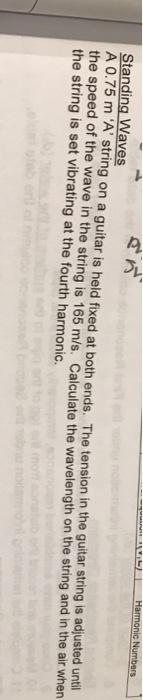Standing Waves A 0.75 m 'A' string on a guitar is held fixed at both ends. The tension in the guitar string is adjusted untl the speed of the wave in the string is 165 m/s. Calculate the wavelength on the string and in the air when the string is set vibrating at the fourth harmonic.

Free Homework App